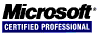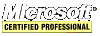# PHP Tips and Tricks

## Numbers

#### Find the Distance Between Two Coordinates

The `get_distance_km` function returns the number of kilometers between two coordinates. Multiply by 0.621 to determine the number of miles.

``````<?php
function get_distance_km(\$latitude1, \$longitude1, \$latitude2, \$longitude2) {

\$distance = acos(sin(\$latitude1) * sin(\$latitude2) + cos(\$latitude1) * cos(\$latitude2) * cos(\$longitude2 - \$longitude1));

if(\$distance < 0) {
\$distance += M_PI;
}

\$distance *= 6378.135;

return \$distance;
}
print get_distance_km(39.50000, -80.0000, 37.0000, -120.00000) * 0.621;
?>
``````

#### Check a Variable for a Number

Use the `is_numeric` function to check if a variable has a number even if the number is a string. If a number has thousands seperators then `is_numeric` returns `false`.

``````<?php
\$num = '123';
if (is_numeric(\$num)) { // return true in this case
print "\$num is a number";
}
?>
``````

#### Determine If Two Floating-Point Numbers Are Nearly Equal

``````<?php
\$delta = 0.00001;
\$i = 9.0000001;
\$j = 9.0000000;
if (abs(\$i - \$j) < \$delta) {
print "\$i and \$j are close enough to be equal";
}
?>
``````

#### Generate Random Numbers Within a Range

``````<?php
\$randnum = mt_rand(65, 97);
print \$randnum;
?>
``````

#### Number Formatting

To print a number with thousands and decimal seperators use the `number_format` function. There are four arguments. The first argument is the number to format, the second specifies the number of decimal places, the third specifies the decimal point character, and the fourth specifies the thousands seperator.

``````<?php
\$num = 1900955343.12345;
\$str = number_format(\$num, 4, '.', ',');
print \$str;
?>
``````

#### Formatting Monetary Values

Use the `NumberFormatter` class.

``````<?php
\$amount = 62345.77;

\$us_formatter = new NumberFormatter('en-US', NumberFormatter::CURRENCY);
\$fr_formatter = new NumberFormatter('fr-FR', NumberFormatter::CURRENCY);

\$us_money = us_formatter->format(\$amount);
\$fr_money = fr_formatter->format(\$amount);

print \$us_money.' '.\$fr_money;
?>
``````

#### Base Conversion

Use the `base_convert` function to convert from one base to another. `base_convert` has three arguments. The first is the number to be converted, the second is the base it is in, and the third is the base you want.

``````<?php
\$hex = 'FE';
\$decimal = base_convert(\$hex, 16, 10);
print \$decimal.' ';

\$binary = '1001011';
\$decimal = base_convert(\$binary, 2, 10);
print \$decimal.' ';

\$decimal = '256';
\$hex = base_convert(\$decimal, 2, 16);
print \$hex;
?>
``````

## Arrays

#### Initialize an Array to a Range of Integers

Use the function `range` to assign a series of consecutive integers to an array. `range` takes three arguments. The first is the start of the range, the second is the end of the range, and the third is how much to increment each integer by.

``````<?php
print '<pre>';
\$arrodd = range(1, 100, 2); // for odd numbers
\$arreven = range(2, 100, 2); // for even numbers
print_r(\$arrodd);
print_r(\$arreven);
print '</pre>';
?>
``````

#### Deleting Elements from an Array

Use the `unset` function to delete one element.

``````<?php
print '<pre>';
\$arr = array(1, 2, 3, 4, 5);
unset(\$arr);
print_r(\$arr);
print '</pre>';
?>
``````

Use the `unset` function to delete multiple noncontiguous elements.

``````<?php
print '<pre>';
\$arr = array(1, 2, 3, 4, 5);
unset(\$arr, \$arr);
print_r(\$arr);
print '</pre>';
?>
``````

Use the `array_splice` function to delete multiple contiguous elements. `array_splice` takes three arguments. The first is the array, the second is the offset, the third is the length. The third argument is optional. Without it, the function removes the offset and all after it.

``````<?php
print '<pre>';
\$arr = array(1, 2, 3, 4, 5);
array_splice(\$arr, 2);
print_r(\$arr);
\$arr = array(1, 2, 3, 4, 5);
array_splice(\$arr, 2, 1);
print_r(\$arr);
print '</pre>';
?>
``````

The output from the above script is as follows:

```Array
(
 => 1
 => 2
)
Array
(
 => 1
 => 2
 => 4
 => 5
)
```

#### Combine Two Arrays into One

Use the `array_merge` function to append one array to another. `array_merge` takes two arguments: the arrays to merge. The return value is the new array. It is also possible to combine two arrays using the plus (+) operator.

``````<?php
print '<pre>';
\$arr1 = array(1, 2, 3, 4, 5);
\$arr2 = array(6, 7, 8, 9, 10);
\$merg = array_merge(\$arr1, \$arr2);
print_r(\$merg);
print_r(\$arr1 + \$arr2);
\$arr1 = array(1 => 'a', 2 => 'b', 'C' => 'c', 4 => 'd', 'E' => 'e');
\$arr2 = array('C' => 6, 7 => 'f', 2 => 8, 0 => 9, 'G' => 'g');
\$merg = array_merge(\$arr1, \$arr2);
print_r(\$merg);
print_r(\$arr1 + \$arr2);
print '</pre>';
?>
``````

The output from the above script is as follows:

```Array
(
 => 1
 => 2
 => 3
 => 4
 => 5
 => 6
 => 7
 => 8
 => 9
 => 10
)
Array
(
 => 1
 => 2
 => 3
 => 4
 => 5
)
Array
(
 => a
 => b
[C] => 6
 => d
[E] => e
 => f
 => 8
 => 9
[G] => g
)
Array
(
 => a
 => b
[C] => c
 => d
[E] => e
 => f
 => 9
[G] => g
)
```

Merging arrays with only numerical keys causes the arrays to get renumbered so values are not lost. Merging arrays with string keys causes the second array to overwrite the value of any duplicate keys. Arrays with both types of keys exhibit both types of behavior.

#### Enlarge an Array

Use the `array_pad` function to grow and array. `array_pad` takes three arguments. The first is the array, the second is the how big the array should grow to, and the third is the value of the new elements.

``````<?php
\$arr = array(1, 2, 3, 4, 5);
\$c = count(\$arr);
print \$c;
?>
``````

#### Converting an Array into a String

Use the `join` function to turn an array into a string. `join` takes two arguments. The first is the delimiter and the second is the array.

``````<?php
\$arr = array('A', 'B', 'C', 'D', 'F');
\$str = join(',', \$arr);
print \$str;
?>
``````

#### Checking an Array for a Key

Use the `array_key_exists` function to know if an array contains a certain key. `array_key_exists` only checks that a key exists in the array, not that there is a value associated with it. Use the `isset` function to determine if a key have a value associated with it.

``````<?php
\$arr = array('z' => 'A', 'y' => 'B', 'x' => 'C', 'w' => 'D', 'v' => 'F');
if(array_key_exists('v', \$arr)) {
if(isset(\$arr['v'])) { print 'key and value exist'; }
}
?>
``````

#### Checking an Array for a Value

Use the `in_array` function to check if an element is in an array.

``````<?php
\$arr = array('z' => 'A', 'y' => 'B', 'x' => 'C', 'w' => 'D', 'v' => 'F');
if(in_array('C', \$arr)) {
print 'value in array';
}
?>
``````

#### Find the Position of an Array's Value

Use the `array_search` to find the position of the value.

``````<?php
\$arr = array('z' => 'A', 'y' => 'B', 'x' => 'C', 'w' => 'D', 'v' => 'F');
\$position = array_search('D', \$arr);
if(\$position !== false) {
print \$position;
}
?>
``````

#### Find the Max or Min Value in an Array

Use the `max` function and the `min` function to find the maximum and minimum values in an array.

``````<?php
\$arr = array('z' => 'A', 'y' => 'B', 'x' => 'C', 'w' => 'D', 'v' => 'F');
\$maximum = max(\$arr);
\$minimum = min(\$arr);
print \$minimum.'-'.\$maximum;
?>
``````

#### Reverse an Array

Use the `array_reverse` function to reverse an array.

``````<?php
\$arr = array(1, 2, 3, 4, 5);
\$rev = array_reverse(\$arr);
print '<pre>';
print_r(\$rev);
print '</pre>';
?>
``````

#### Sorting Parallel Arrays

Use the `array_multisort` function to sort parallel arrays.

``````<?php
\$numbers = array(5, 2, 10, 4, 0);
\$colors = array('blue','yellow','aqua','green','white');
array_multisort(\$numbers, \$colors);
print '<pre>';
print_r(\$numbers);
print_r(\$colors);
print '</pre>';
?>
``````

The output of the previous script is as follows:

```Array
(
 => 0
 => 2
 => 4
 => 5
 => 10
)
Array
(
 => white
 => yellow
 => green
 => blue
 => aqua
)
```
<< < [Page 2 of 6] > >>